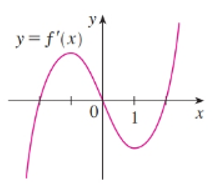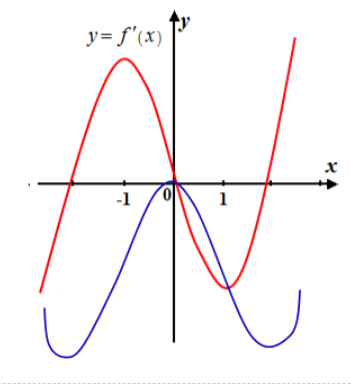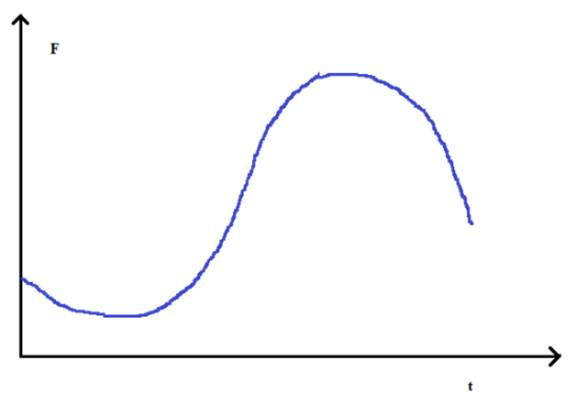# The intervals in which f increases or decreases.### Single Variable Calculus: Concepts...

4th Edition
James Stewart
Publisher: Cengage Learning
ISBN: 9781337687805### Single Variable Calculus: Concepts...

4th Edition
James Stewart
Publisher: Cengage Learning
ISBN: 9781337687805

#### Solutions

Chapter 2, Problem 43RE

(a)

To determine

## To find:The intervals in which f increases or decreases.

Expert Solution

The function f is increasing in intervals (2,0) and (2,) , and decreasing in intervals (,2) and (0,2) .

### Explanation of Solution

Given information:

The given graph is shown in figure ().Figure (1)

Calculation:

If f'(x)>0 on an interval, then the f is increasing on that interval.

If f'(x)<0 on an interval, then the f is increasing on that interval.

From the above graph, the function f is increasing in intervals (2,0) and (2,) , and decreasing in intervals (,2) and (0,2) .

f is increases when f'(x) is positive and decreases when f'(x) is negative.

Therefore, the function f is increasing in intervals (2,0) and (2,) , and decreasing in intervals (,2) and (0,2) .

(b)

To determine

### To find:The values of x does f have a local maximum and minimum.

Expert Solution

The f have a local value maximum is x=0 and minimum value is x=2,x=2 .

### Explanation of Solution

Given information:

The given graph is shown in figure ().Figure (1)

Calculation:

The point at which f'(x) changes from negative to positive is the local minimum point at the point at which f'(x) changes from positive to negative is the local maximum point.

Here, at x=2 and x=2 , f'(x) changes from negative to positive and at x=0 , f'(x) changes from positive to negative.

f have a local minimum value is x=2,x=2 and the value of x for which f have a local maximum value is x=0 .

Therefore, the f have a localvalue maximum is x=0 and minimumvalue is x=2,x=2 .

(c)

To determine

### To find:Where is f concave upward or downward.

Expert Solution

The f is concave upward in (,1) and (1,) , concave downward in (1,1) .

### Explanation of Solution

Given information:

The given graph is shown in figure (1).Figure (1)

Calculation:

The function f is concave upward when f'(x) is increasing and f is concave downward when f'(x) is decreasing.

Hence, f is concave upward in (,1) and (1,) , concave downward in (1,1) .

Therefore, the f is concave upward in (,1) and (1,) , concave downward in (1,1)

(d)

To determine

Expert Solution

### Explanation of Solution

Given information:

The given graph is shown in figure (1).Figure (1)

Graph:

From the given figure that f'(x) is negative when <x<2 and 0<x<2 . So, the original function f must be decreasing on the interval (,2) and (0,2) .

Similarly,

f'(x) is positive when 2<x<0 and 2<x< . So, the original function f must be increasing on the interval (2,0) and (2,) .

It is known that f(0)=0 , so, we draw the graph of f at a given point (0,0) .

The graph for the function f is shown in figure (2).Figure (2)

The rough sketch of graph F as a time t is shown in figure (3).Figure (3)

Interpretation: Graph for the function f is shown in figure (2).

### Have a homework question?

Subscribe to bartleby learn! Ask subject matter experts 30 homework questions each month. Plus, you’ll have access to millions of step-by-step textbook answers!